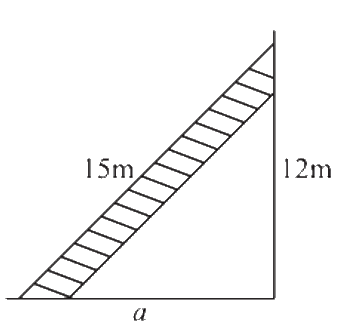# Ex.6.5 Q3 The Triangle and Its Properties - NCERT Maths Class 7

Go back to  'Ex.6.5'

## Question

A $$\rm{}15\, m$$ long ladder reached a window $$\rm{}12\, m$$ high from the ground on placing it against a wall at a distance $$a$$. Find the distance of the foot of the ladder from the wall.Video Solution
Triangle & Its Properties
Ex 6.5 | Question 3

## Text Solution

What is  known ?

Length of ladder is $$\rm{}15\, m$$ and the height of the window from the ground is $$\rm{}12\,m.$$

What is unknown?

The distance of the foot of the ladder from the wall.

Reasoning:

This question is based on the concept of right – angled triangle. As it is clear from the figure
that the ladder is kept slanted on the wall so, consider length of the ladder as hypotenuse
i.e.: $$AB =\rm{}15\,m$$ and as it is kept slanted on the wall so, we consider wall as perpendicular
i.e.: $$AC = \rm{}12\, m.$$ Now, you must find out the distance of the foot of the ladder from the wall
i.e.: $$BC = a.$$ Now by applying Pythagoras theorem in triangle $$ABC,$$ we can find out $$BC.$$

For better visual understanding draw a right-angled triangle consider ladder as hypotenuse, wall as perpendicular and distance between the foot of the ladder and wall as base.

Steps:

Given, Length of ladder $$AB = \rm{}15\, m$$

Length of wall $$AC = \rm{}12\, m$$

To find $$(BC) =$$ distance of the foot of the ladder from the wall.

According to Pythagoras theorem,

\begin{align}&{{\left(\text{Hypotenuse} \right)}^2} \\ &= {\rm{ }}{{\left(\text {Perpendicular} \right)}^2} + {\rm{ }}{{\left( \text{Base} \right)}^2} \end{align}

\begin{align} {{\left( {AB} \right)}^2} &= {{\left( {AC} \right)}^2} + {{\left( {BC} \right)}^2}\\{{\left( {15} \right)}^2} &={{\left( {12} \right)}^2} + {{\left( a \right)}^2}\\225 &= 144 + {{\left( a \right)}^2}\\225-144 &= {{\left( a \right)}^2}\\81 &= {a^2}\\a &= 9 m\end{align}

Therefore, the distance of the foot of the ladder from the wall is $$\rm{}9 \,m.$$

Useful Tip:

Whenever you encounter problem of this kind, it is better to understand its visually.

Learn from the best math teachers and top your exams

• Live one on one classroom and doubt clearing
• Practice worksheets in and after class for conceptual clarity
• Personalized curriculum to keep up with school